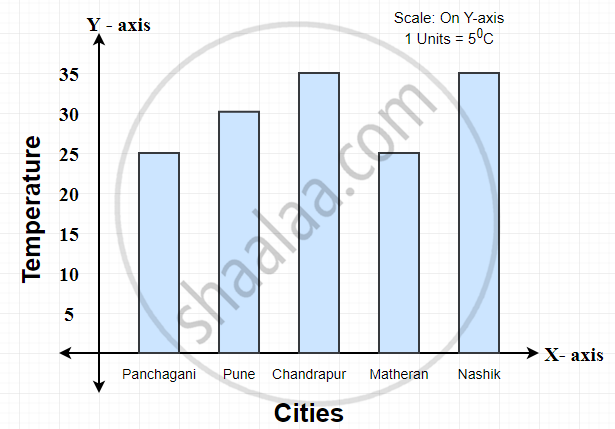# Interpretation of Bar Graphs

#### definition

Interpretation of a bar diagram: Interpretation of a bar diagram means the action of explaining the meaning of the bar diagram.

# Interpretation of bar diagrams:

Interpretation of a bar graph means the action of explaining the meaning of a bar diagram.

1) This bar graph shows the maximum temperatures in degrees Celsius in different cities on a certain day in February.

Observe the graph and answer the questions.1. What data is shown on the vertical and the horizontal lines?

2. Which city had the highest temperature?

3. Which cities had equal maximum temperatures?

4. Which cities had a maximum temperature of 30°C?

5. What is the difference between the maximum temperatures of Panchgani and Chandrapur?

### Solution:

1. Cities are shown on horizontal lines (i.e., X-axis), and temperatures shown on vertical lines (i.e., Y-axis).
2. Chandrapur and Nashik cities had the highest temperature.
3. Chandrapur and Nashik cities had equal maximum temperatures.
4. Chandrapur and Nashik cities had a maximum temperature of 30°C.
5. The difference between the maximum temperatures of Panchgani and Chandrapur is 10°C.
If you would like to contribute notes or other learning material, please submit them using the button below.

### Shaalaa.com

How To Interpret A Bar Graph? [00:06:48]
S
0%